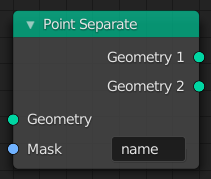# Point Separate¶The Point Separate node.

The Point Separate node produces two geometry outputs. Based on the Threshold and the input Attribute, the point cloud component of the input geometry is split between the two outputs.

Tip

This node can be combined with the Attribute Compare node for a more precise control of which points are separated to a given output geometry.

## Inputs¶

The name of the attribute used to calculate which geometry output each point will belong to. Any value of “true” will move to the second output, and any value of “false” will move the point to the first output.

If the attribute has any data type besides Boolean, the value will be implicitly converted, so a value of exactly zero is false, and any other value is true.

## Outputs¶

Geometry 1

Points with a mask attribute value of “false” will be moved to the first output.

Geometry 2

Points with a mask attribute value of “true” will be moved to the second output.